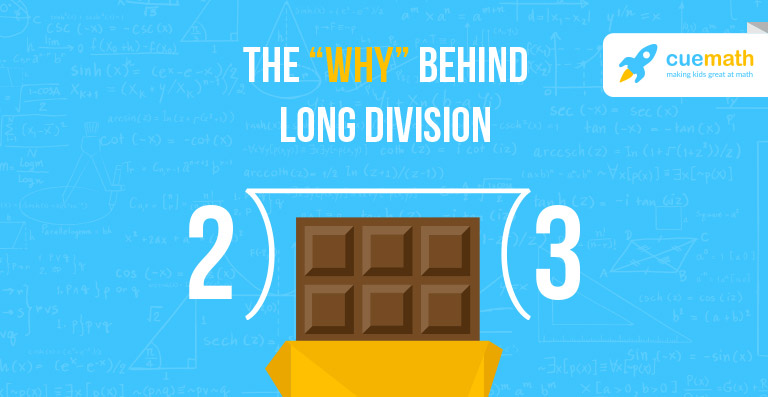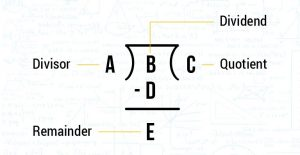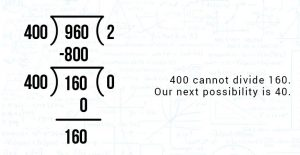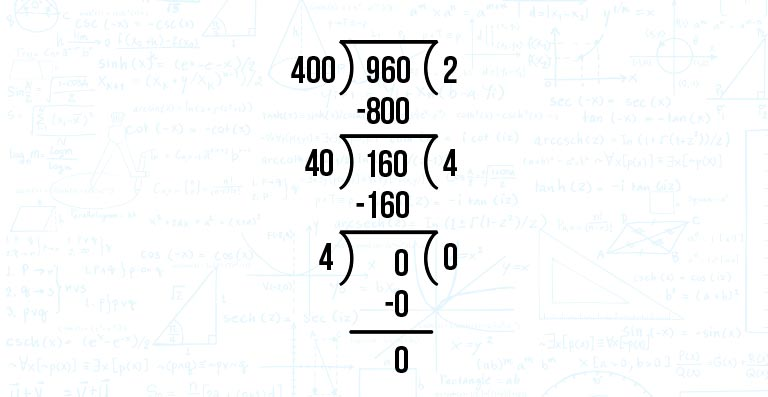# WHY DOES LONG DIVISION WORK?

Go back to  'Math Concepts'Students are first introduced to the concept of multiplication through the multiplication tables, then direct questions and statement problems. Later on, they are taught the concept of division which they mug up.

Eventually, when both the concepts are integrated, they get confused.

Long division is one such method which makes use of the concepts of division, multiplication and subtraction. With these many basic concepts integrated, they are bound to face some difficulty while learning it.

Instead of just learning about division, students should understand the use of division, the meaning of divisors, dividends and multiples.

In this article, we will show you why the long division method works and how multiplication complements the process.

What is division?

Division is repeated subtraction. It is the process of dividing a number (dividend) with another number with the same or lesser value (divisor) to find the quotient and a remainder. This remainder is 0 if the divisor is a factor of the dividend, it’s non-zero if the divisor is not a factor.

If the quotient is later multiplied with the divisor, we get the dividend.Why does it work?

In order to see why the long division method works, let’s take an example. Let’s say there are 960 sweets that need to be boxed. Each box can contain only 4 sweets, how many boxes would we need?

Now, we don’t have to subtract 4 from 960 continuously to arrive at the answer.

The long division method will help us reach the answer. Since 9 is at the hundreds place in 960, we consider 400 instead of 4 so that 4 is also in the hundreds place..

400 would go twice in 960. It’s easier if we check “How many times would 4 go in 9?” The answer to that would be 2.

This would be the first digit of the quotient.

When we subtract 800 from 960, we get the first remainder as 160.The next step would be to gauge how 400 would divide 160. This is not possible as 400 is greater than 160 and so we consider 40.

40 would go 4 times in 160.

The second digit of the quotient will therefore be 4.

160 is wholly divisible by 40 and leaves no remainder.In the end, 4 goes 0 times in 0 which is how we get the last digit of the quotient. The quotient obtained from this calculation is 240 as seen on the right side of the image above.

So there, you know WHY the long division method works. Don’t you think you’re better equipped to help your students out now?

0